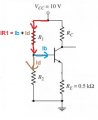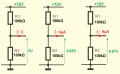# Find the voltage gain

Joined Feb 3, 2016
42
OK I see. This formula "Rth=0.1(1+β)Re" is a rule of thumb used sometimes by a designer. And this rule means that we must choose R1||R2 < 0.1(1+β)Re for "bias-stable". This rule ensure that our voltage divider voltage is "stiff". Because base current is a load for our voltage divider. And to ensure that our divider voltage do not change much after we loading it we use this "rule of thumb" to select R1 and R2. The slightly different rule of thumb is to choose voltage divider current Id = Vcc/(R1+R2) at least 10 times the base current. So we have:
R1 = (Vcc - Vb)/(11*Ib)
R2 = Vb/(10*Ib)
I probably understand it.
But how to get these two equations?
R1 = (Vcc - Vb)/(11*Ib)
R2 = Vb/(10*Ib)

#### Jony130

Joined Feb 17, 2009
5,405
Now in order to make the resistor voltage divider "stiff" you need to make sure that the unloaded current through the resistors (call it Id ) is at least 10 times the load current (in this case IB is the load for the voltage divider). Otherwise the load current draws too much current away from R2, which causes the voltage at the output of the voltage divider decrease too much. So to ensure this "stiff" voltage we have to choose R1 and R2 in this way:
The voltage drop across R1 is equal to V_supply (Vcc) minus the Voltage at the transistor base (Vb).
So from the Ohms law we have
R1 = V/I = (Vcc - Vb)/I
And the R1 resistor current is equal to Id + Ib where Id = 10*Ib so,we have 10*Ib+Ib = 11*Ib.
And R2 is simply Vb/Id = Vb/(10*Ib)Joined Feb 3, 2016
42
what does "stiff " mean?
Is the stiff voltage divider mean that the base current is much smaller than the current entering R2 ?

#### Jony130

Joined Feb 17, 2009
5,405
what does "stiff " mean?
stiff voltage----> - Voltage divider output voltage is independent of the load current
Is the stiff voltage divider mean that the base current is much smaller than the current entering R2 ?
No, to make voltage divider "stiffer" we select divider current much larger than the base current.
Example 1:
"Poor" voltage divider Id = 2* Iload_maxA "stiffer" voltage divider Id = 10*Iload_max•